﻿ New Perspective of Expanding Universe and Estimate for Mass of Universe by New Gravity EquationInternational Journal of Astronomy

p-ISSN: 2169-8848    e-ISSN: 2169-8856

2017;  6(1): 17-19

doi:10.5923/j.astronomy.20170601.03### New Perspective of Expanding Universe and Estimate for Mass of Universe by New Gravity Equation

Visakhapatnam, India

Correspondence to: Siva Prasad Kodukula, Visakhapatnam, India.
 Email: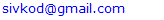Abstract

It is an application of a new classical equation for gravity which is applicable even in plank scale with a new parameter Siva’s constant ‘K’. The result concluded a new perspective of ‘Expanding Universe’ and says that the universe is not expanding. All the observations will show that the universe is expanding due to the consideration of constant ‘rest mass’ throughout the expansion. The constant ‘rest mass’ has been estimated by new gravity equations. It is numerically equal to the some of the earlier estimates concluded by other methods.

Keywords: Mass of Universe, Siva’s constant ‘K’, New gravity equation, Expanding universe, Plank scale

Cite this paper: Siva Prasad Kodukula, New Perspective of Expanding Universe and Estimate for Mass of Universe by New Gravity Equation, International Journal of Astronomy, Vol. 6 No. 1, 2017, pp. 17-19. doi: 10.5923/j.astronomy.20170601.03.

### Article Outline

1. Introduction
2. Calculation & Discussion
3. Conclusions

### 1. Introduction

The physical interpretation of inertial frame of reference and its change has been explained as force of gravitation with an absolute velocity ‘V’ and follows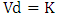as explained in previous papers . In this process it is concluded that there will exist two forces obeys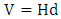andand explained as expansion and gravitational contraction respectively. Where ‘H’ is Hubble’s constant and ‘K’ is Siva’s constant. The universe at any stage with diameter ‘d’ will have both the forces in opposite directions. When the universe reaches its maximum diameter, the velocity ‘V’ will be equal to velocity of light ‘c’.
After next ‘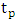’ seconds it will be with ‘plank length’ ‘’.
Thus this is the final diameter in expansion and commencement of gravitational collapse and contraction. This change is a natural phenomenon of space time and follows law of observation. A new gravitational equation  has been introduced to explain gravitational force with respect to this phenomenon in the previous paper . It has been utilized for calculations and discussions. The new equation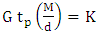along withan equation for gravity with absolute velocity ‘V’ as explained in previous papers [1, 2] has been used for the estimate of mass of Universe.
The result is numerically equal to some of the earlier estimates made by other researchers [3, 4].

### 2. Calculation & Discussion

Sivas gravity equation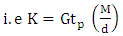(1)
Where ‘G’ is Newton’s gravitational constant
‘K’ is Siva’s constant’ is plank time
‘M’ is mass of the body
‘d’ is the distance between two points in the space time associated to mass ‘M’.
We have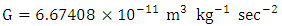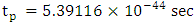If we consider it for whole universe,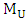is mass of Universe and ‘d’ is Hubble’s diameter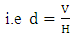(2)
Where
Hubble’s constant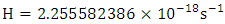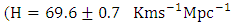The length of one parsec  is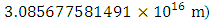‘V’ is the velocity at a distance ‘d’ as per Hubble’s law.
Substitute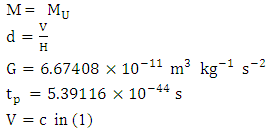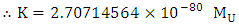(3)
We have gravity equationAt this stage ‘V’ will be minimum
We can calculate that minimum velocity ‘V’ by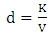(4)
Substitute ‘d’ of (4) in (1)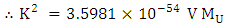(5)
We have plank length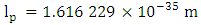Plank mass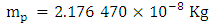Substitute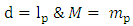in (1)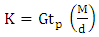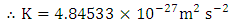(6)
Units of dimension will be changed wherever we use the equation.
The numerical value remains constant.
Substitute this K value in (3)
Total mass of the universe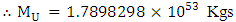Substituteand K value in (5)
Final velocity of expansion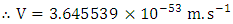(7)
The physical meaning is-
If values of K andare constants, the distance‘d’ will be different with different velocity of expansion or contraction.
If K is constant, the ratio ofand ‘d’ will be constant. Butwill change with ‘d’.
Therefore,
To satisfy both the points K andmust be constant at any stage of universe and there exist a specific velocity at a distance ‘d’ viewed as expanding universe.
Here if we won’t consider the expansion, there will not be any variation in ‘d’ and concerned ‘V’.
Thus the diameter ‘d’ of total mass of universe= Mass of the Universe with ‘plank mass’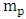and diameter as plank length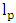to keep K as constant.
Both the cases are same. For both the cases K will be constant but there will be a variation in mass of the universe in non expansion case. If mass is constant all the observations will show that the universe is expanding. The expansion and non expansion are same when we consider the mass variation. Mass variation is an affect of variation in its own space time density. Here, it is to be noted that the density of mass is different from its space time density associated to that mass. If we consider that there is no variation in space time density, there will not be variation in mass and it remains constant throughout the expansion. If mass is constant and the diameter of the universe changes then the result of observation is ‘expanding Universe’ for which ‘K’ is constant.
Mathematically,
we have two equations (1) and (3)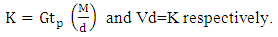In order to keep both K and M as constants and to satisfy both the equations the d must be associated with a velocity and as the ‘d’ changes with velocity, there exist a change of velocity with ‘d’ and viewed as a force of expansion. The same will be applicable for contraction also.
Thus we can conclude that the expansion of universe is a considerable phenomenon in the aspect of observation. The Universe is not at all expanding or contracting. It remains as it is. The concept of observation has to be reconsidered on fundamental basis.
In the aspect of expanding universe concept, the final velocity of expansion at far end of Hubble Universe reaches light velocity, the force of gravity will be weak and the expansion will be halted. But the velocity will not be zero. It will be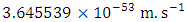. This velocity will gradually increase towards the gravitational collapse.

### 3. Conclusions

1. The application of Siva’s gravity equation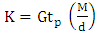to the universe estimated the mass of universe as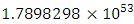Kgs. which is coinciding with one of the earlier estimates made by other researchers [3, 4].
2. The universe is expanding as per physical observations defined by present laws of physics. In another perspective of observation the universe is not expanding. It is a change in space time density related to a new parameter termed as Siva’s Constant ‘K’.
3. In the perspective of the expanding universe concept, the estimated mass of the universe is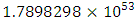Kgs the final velocity of the expanding universe is calculated as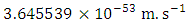. After this stage, gravitational collapse will start.

### References

  Kodukula, S.P., 2017, Role of Observer & Consciousness on Special Theory of Relativity and its Influence on Kinetic Energy, International Journal of physic, 5(4), pp. 99-109. DOI:10.12691/ijp-5-4-1. http://pubs.sciepub.com/ijp/5/4/1.  Kodukula, S.P., 2017, Importance of Siva’s Constant ‘K’ in Redefining Law of Gravitation as an Affect of Consciousness, International Journal of Theoretical and Mathematical Physics, 7(6): 155-158. DOI: 10.5923/j.ijtmp.20170706.01. http://article.sapub.org/10.5923.j.ijtmp.20170706.01.html.  Carvalho, Joel C., 1995, Derivation of the Mass of the Observable Universe, International Journal of Theoretical Physics, 34(12), p. 2507.  Claude Mercier., 2015, Calculation of the Apparent Mass of the Universe. Web site: Hypotheses and reflections on the universe, Available on line: www.pragtec.com/physique/ (accessed on 14 December 2017).  Peter J. Mohr, David B. Newell, Barry N. Taylor, 2015, CODATA Recommended Values of the Fundamental Physical Constants: 2014. https://arxiv.org/pdf/1507.07956.pdf.  C. L. Bennett, D. Larson, J. L. Weiland, and G. Hinshaw, The Astrophysical Journal, 794:135 (8pp), http://iopscience.iop.org/article/10.1088/0004-637X/794/2/135/pdf doi:10.1088/0004-637X/794/2/135.  Andrej Prˇsa, Petr Harmanec, Guillermo Torres, Eric Mamajek, Martin Asplund, Nicole Capitaine, Jørgen Christensen-Dalsgaard, ´Eric Depagne, Margit Haberreiter, Saskia Hekker, James Hilton, Greg Kopp, Veselin Kostov, Donald W. Kurtz, Jacques Laskar, Brian D. Mason, Eugene F. Milone, Michele Montgomery, Mercedes Richards, Werner Schmutz, Jesper Schou and Susan G. Stewart, Nominal values for selected solar and planetary quantities: IAU 2015 Resolution B3, arXiv:1605.09788v1 [astro-ph.SR], 31 May 2016. http://www.iau.org/static/resolutions/IAU2015English.pdf.